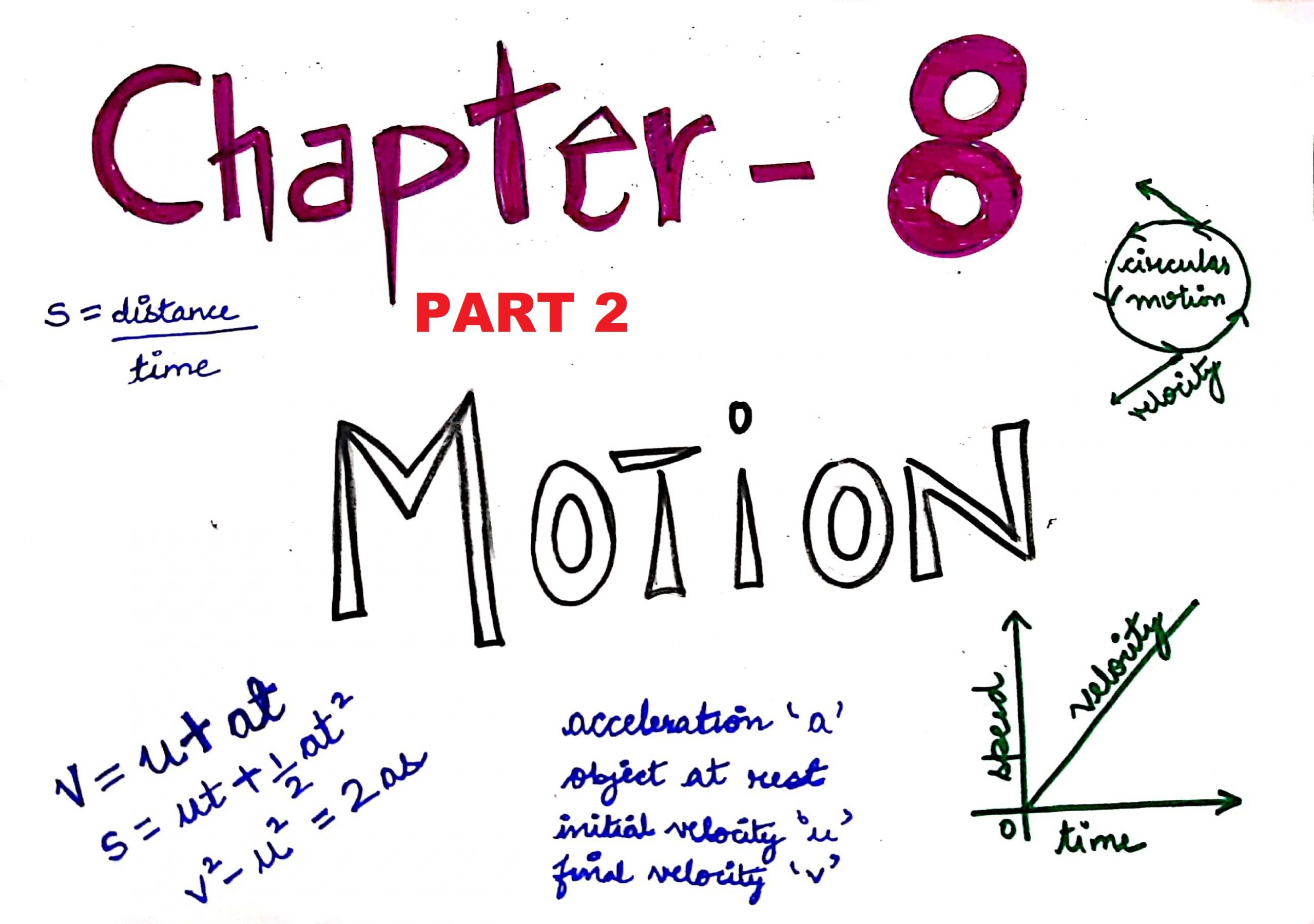# NCERT solutions for class 9 science chapter 8 motionNcert solutions for class 9: Motion is related to the movement of any object. Mechanics is the branch of physics that deals with Motion of a body.

Before learning more facts about motion let us first understand what actually motion is:

Motion:

An object is said to be in motion when it changes its position with respect to the surrounding at a given period of time

Rest :

An object is said to be in when it does not change its position during a time

## Different types of motions are :

Linear motion : straight line motion

Circular motion: In circle format

oscillatory /vibratory : to and fro motion

Now let us move towards the important keywords. We will be using these terms frequently :

1. Scalar : This is a type of physical quantity having only magnitude and no directions
2. Vector : These are the physical quantities having magnitude and directions as well.

3. Distance : The actual length of the path travelled by an object during its journey from initial or starting point to final point is called distance travelled.Magnitude of distance can never be zero or negative and the SI unit of distance is metre, Distance is a scalar quantity (only magnitude),

4. Displacement : Displacement is the length of the shortest path between the initial and the final point. The magnitude of displacement can be zero and negative when the initial and final point coincide. The S.I unit of displacement is also metre- Displacement is a vector quantity(both magnitude and directions), Displacement will always be equal to less than the distance travelled.

Types of motion:

Uniform motion: When a body travels equal distance in equal interval of time.

it is said Uniform motion. The Time and Distance graph of uniform motion

Non-Uniform Motion : When the body cover unequal distances in equal time intervals.

The graph of this motion is curved line.

Non-uniform motion is further divided into:

Accelerated and De -accelerated motion

Now let us understand some facts about speed and other terms related to it.

Speed — We can get speed by the ratio of Distance and Time. It’s S.I unit is m/s.

the Speed (s) = Distance (d)/Time (t)

for Speed is the measure of total distance covered per unit time.

get Speed can be uniform or non-uniform.

Average Speed = Total distance travelled / total time taken

=(d1+d2+d3+dn) / (t1+t2+t3+tn)

## Velocity

It is the measure of speed or change in speed in specific unit of time. It is a vector quantity.

Mathematically , velocity = displacement / time

Velocity is a vector quantity and its S.I. unit is m/s. Average displacement will be calculated as-

Average velocity =  (initial velocity + final velocity) / 2 = (u+v) / 2

OR  = total displacement / total time

Velocity can be positive, negative or zero

Acceleration:

It is the rate of change of velocity per unit time. It is observed in non- uniform motion. Mathematically, acceleration = final velocity – initial velocity / time

= (v-u) / t

If acceleration “a” is positive then it symbolizes that speed/velocity is increasing with time,

If acceleration is negative, it is called retardation and symbolizes that speed/velocity is decreasing with time

Speed vs time graph for uniform motion and non – uniform motion.

2.  Speed vs Time graph for body at rest: